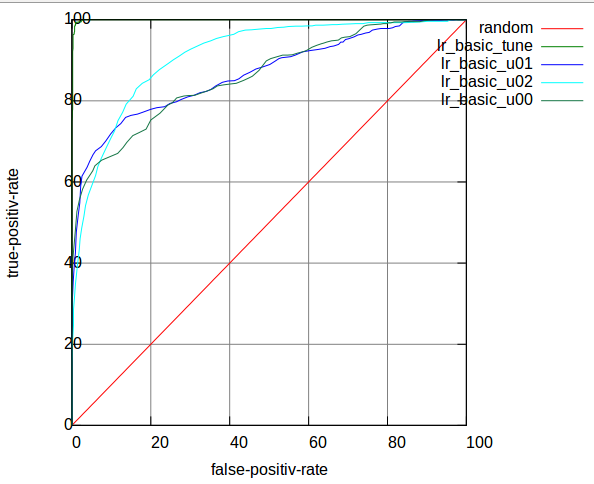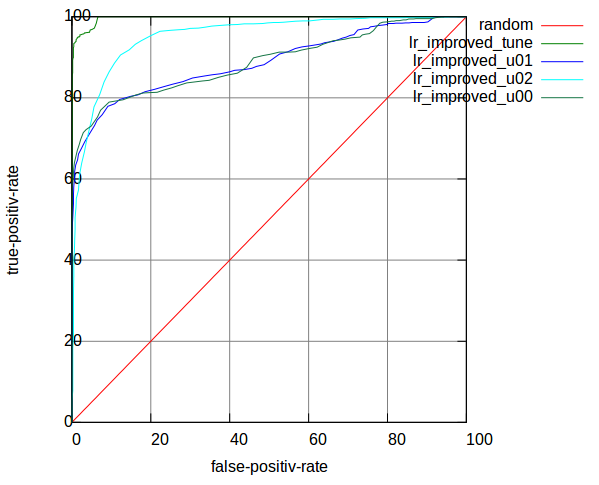Spam Filter (3) -- Logistic Regression & TF-IDF

I studied this method from one of papersposted on Third Conference on Email and Anti-Spam (CEAS 2006).  I implemented the basic Logistic Regression (LR) method followed by the conduct of it and it worked as good as the basic implementation of Graham’s Method.

Concepts

I will learn a set of weights for each word in the body or header of the message. When a new message arrives, I find this list of words, and sum the weights associated with those words. $P(Y=spam | \vec{x}) = \frac{exp (\vec{w} * \vec{x})}{1 + exp (\vec{w} * \vec{x})}$ , where $$\vec{w}$$ is the vector of weights, and $$\vec{x}$$ is a vector of 1’s or 0’s with a 1 in the position corresponding to each word.

The equation results a probability between 0 and 1. If the probability is over some threshold, I predict that the email is spam; otherwise predict the email is ham.

So, this is the equation I will use to evaluate how much is the probability of a new mail to be Spam. A similar prediction as Naive Bayes Filter. And then I will learn the weights of every word in training process.

The actual training algorithm is very simple. Given as following:

$$\vec{w}$$ = 0 // initialize weights to 0
for each $$\vec{x_i}, y_i$$
$$P = exp⁡(\vec{x_i} * \vec{w}) / (1 + exp⁡(\vec{x_i} * \vec{w}))$$
if ($$P$$ > 0.5)
predict Spam;
else
predict Non-spam;
if ($$y_i$$ == 1 )
$$w ̅= w + (1 - P) * x_i * rate$$
else
$$w ̅= w - P * x_i * rate$$

Suppose threshold is 0.5 here; learning rate makes sure that the step taken in the direction of the gradient is not too large.

Implement Basic Logistic Regression Filter

I implemented Basic Logistic Regression method in three steps.

• Step 1. Learn $$w$$on train_data;
• Step 2. Evaluate each mail based on $$P(Y=spam | \vec{x}) = \frac{exp (\vec{w} * \vec{x})}{1 + exp (\vec{w} * \vec{x})}$$ , so that I could get probability of a mail being Spam given words vector x ̅ of this mail;
• Step 3. The parameter tuning and train to evaluate process are similar to that of Bayesian Filtering.

The basic result is shown in Table 4:

Table 4: AUC of Basic LR

tune u01 u02 u00_tune u00
AUC 0.999212 0.867380 0.912238 0.999411 0.848224
u00_tuned 0.999588 0.862130

Figure 6 shows ROC curves for Table 4:Figure 6: ROC curves of Basic LR

Improve Basic Logistic Regression: re-modified TF-IDF

Logistic Regression with TF-IDF is a common technique in text classification. TF-IDF is generally used in search engine. It is a numerical statistic which reflects how important a word is to a document in a collection.

First Attempt: Default TF-IDF

I learned how to use TF-IDF  to restructure the weight vector $$\vec{w}$$ of each word.

$$\text{TF}_{t,d}$$ (Term Frequency) = frequency of a term t in document d / total words count (or most frequent words count) in document d;

$$\text{IDF}_t$$ (Inverse Document Frequency) = $$\log⁡( \frac {\text{Corpus size}} {\text{Document count contains term }t + 1} )$$

$$\text{TF-IDF} = \text{TF}_{t,d} * \text{IDF}_t$$ , it can evaluate how important a word is in a mail.

Then, the weight of a word can be reconstructed as following formular: $W_i = \frac {tf_i * \log{(M / f_i)}} {\sqrt{ \sum_{j=0}^N (tf_j * \log{(M / f_j)})^2 } } * W_i$ , where $$W_i$$ is the vector of weights, $$\text{TF}_i$$ is term frequency of word i in the e-mail, $$M$$ is the total number of the email collection, $$N$$ is the total number of the words in a detailed email.

It also takes the importance of each word into consideration onto weight, which is similar to the method used for picking most interesting words in Bayesian Filter.

However, this attempt failed for even decreasing the AUC results.

Second Attempt: modified TF-IDF

Limitation of traditional TF-IDF: If a word has high term frequency (TF) in both Spam and Non-spam, it does not have good discriminant validity. This also happens in inverse document frequency (IDF). 

Improved TF-IDF: $W_i = \text{TF}_i * \text{IDF}_i = \log{(| Sf_i - Hf_i |)} * (| \log{(S / n_i)} - \log{(H / n_i)} |)$, where $$Sf_i$$ is the ith word term frequency in Spam, and $$Hf_i$$ is the ith word term frequency in Healthy. $$S$$ is total number of Spam, $$H$$ is total number of Healthy.

By evaluating the difference of word’s TF and IDF between Spam and Non-spam, improved TF-IDF can better show the word’s discriminate validity, especially the low frequency words.

But these two attempts had not worked well, they even decreased the AUC in u02.

See the result of second attempt in Table 5:

Table 5: AUC of modified TF-IDF LR

u00 u01 u02
AUC 0.859492 0.867861 0.847229

Third Attempt: re-modified TF-IDF

The second attempt sound reasonable, it should have taken effect. But it has missed some important concept in the original TF-IDF. It has not taken the evaluate mail itself into consideration. $\begin{eqnarray}\text{Simple TF}_t & = & \frac {f_{t,d}} {Max(f_{i,d})} \\ \text{Modified TF}_t & = & \log{|Sf_t - Hf_t |} \\ \text{Re-modified TF}_t & = & \frac {\log{|Sf_t - Hf_t |}} {Max(f_{i,d})} \end{eqnarray}$ , where I added the denominator here, to taken the feature of an evaluate mail into consideration. This increased the AUC decently.

Table 6 shows the result of Re-modified TF-IDF with Logistic Regression Filter:

Table 6: AUC of Re-modified TF-IDF on LR

tune u01 u02 u00_tune u00
AUC 0.999371 0.887771 0.952392 0.999475 0.881424
u00_tuned 0.999606 0.888191

The ROC curves of Re-modified TF-IDF on LR are shown in Figure 7:Figure 7: ROC curves of Re-modified TF-IDF on LR

 Online discriminative spam filter training] (2006) Joshua Goodman, Wen-tau Yih. The Conference on Email and Anti-Spam 2006

 Study on applications of spam filtering based on logistic regression 基于Logistic回归的垃圾邮件过滤应用研究 (2008) Wang Qing-xing. Zhejiang University, China

 Filtering Chinese Spam Email Using Logistic Regression 基于Logistic回归的中文垃圾邮件过滤方法 (2008) Wang Qin-xing, Xu Cong-fu, He Jun. Zhejiang University, China

 Application of TF-IDF and Cosine Similarity] (2013) Ruan Yi-feng
 Tweet